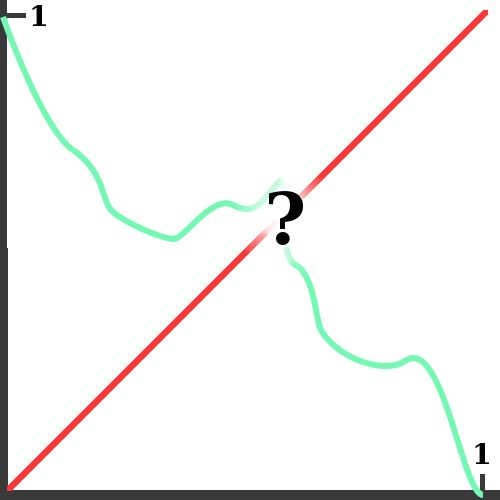# Fun Topics in Calculus

Some of the things we cover in calculus are related to much richer topics. I have a selection of these here, together with some links for the curious. You might also be interested in Fun Topics in Calculus II.

## The Intermediate Value Theorem ¶The intermediate value theorem guarantees that the green curve must cross the red line. But it doesn't say where!

Most of us feel (at least initially) like the intermediate value theorem is pretty obvious. But we've also seen in class that it can have surprising and far-reaching consequences. One of these is that you can always stabilize a level chair (or table) on an uneven floor: here is a mathematical article describing the precise result, known sometimes as the Wobbly Table Theorem. And here is a nice video of a table turning on a wavy surface. By the intermediate value theorem, there must be some configuration where the table doesn't wobble! In February of 2007, NPR Weekend Edition ran a short segment about this called The Science of Steadying a Wobbly Table!

Here's another fun problem which you can solve with the intermediate value theorem; I found it at the eon blog, although apparently it's been around for a while.

A monk starts to climb a mountain at 8:00 am and reaches the summit at noon. He spends the rest of the day and that night on the summit. The next morning he leaves the summit at 8:00 am and descends by the same route he used the day before, reaching the bottom at noon. Prove that there is time between 8:00 am and noon at which the monk was at exactly the same spot on the mountain on both days. Note that the monk can walk at different speeds, rest, or even go backward whenever he wants.

This type of problem is known as a fixed-point problem (Wikipedia), and they have all kinds of applications.

## Elliptic Curves ¶An elliptic curve; in this example, a = -1 and b = 1, from Wikimedia Commons.

When we learned about implicit differentiation, we learned how to find horizontal and vertical tangents for curves like the one pictured here. This is an example of an elliptic curve (Wikipedia). Elliptic curves have a number of fascinating algebraic and geometric properties: for example, every line through an elliptic curve crosses the curve exactly 3 times (if you count the crossings right). These curves have been appreciated for their beauty and structure for centuries. More recently, they are appreciated for their applications to cryptography (Wikipedia).

## Newton's Method and Fractals ¶A fractal generated by Newton's Method for the function z^3 - 1 in the complex plane.

Newton's Method (Wikipedia) is a great way to find roots of polynomials, or other kinds of functions. Depending on what your starting point is, the method might converge to any of the zeroes of the function. It can be very hard to tell which root the method will converge to, as illustrated by the picture at right. If you start at a blue point, the method will converge to (1,0); if you start at a red point, the method will converge to (-1/2, sqrt(3)/2); if you start at a green point, the method will converge to (-1/2, -sqrt(3)/2). But the green, red, and blue regions are arranged in a strange, interesting pattern. Patterns like this are called fractals (Google images).

## Random Walk in 2D and 3D ¶

Some LOGO code showing (sort of) the difference between random walks in 2D and 3D--think of the line color as *the third dimension!* Can you see the qualitative difference between the 2D path and the 3D path? (Hint: does the 3D path return to itself as often as the 2D one?) This quality has to do with what probabilists call recurrence and transience. The picture at right links to a nice javascript LOGO interpreter and the code to produce pictures like this.The 'color-axis'

To show the 'color-axis', the turtle makes a spiral while moving constantly in the 'color' direction. As you can see, we run out of colors before too long and so have to repeat.

If you like, here is a text file of the LOGO code.

## Platonic Solids and Euler Characteristic

You can use the Euler Characteristic to see why there can only be 5 Platonic solids. If this comes up in class, maybe I'll explain it here.

tags: fun-topics

## Site InfoThe content of nilesjohnson.net is licensed under CC BY-NC 4.0.